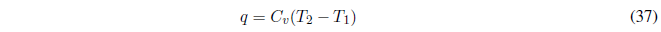AV constant the work done is null $w=0$.

Therefore, $\Delta U=q$
The heat is calculated with the relationship:If the heat capacity is constant, the integral gives us:The calculation of the enthalpy change is done with: $\Delta H=\Delta U + V\Delta P$